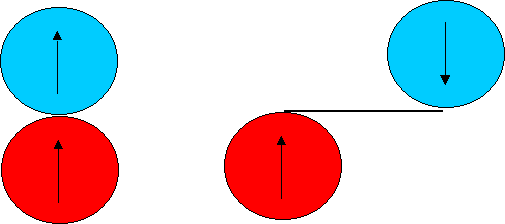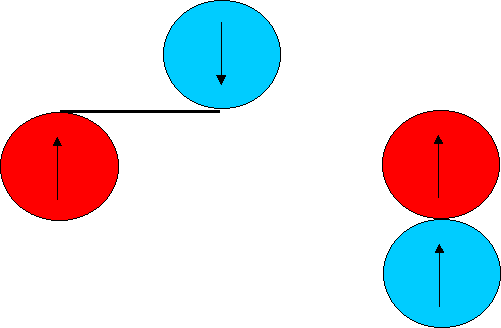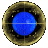Process Approach to Coin Dragon

The Process Heuristic is a bundle of problem solving ideas based upon the following scheme:
Look on the situation as a change from initial state A to final state B. Invent some fictitious state C, for which changes from A to C and from C to B can both be easily found.

The blue coin has to roll a distance equal to half its circumference about the red coin. Let's do this via a fictitious intermediate stage:

Roll the blue coin horizontally a distance of half of its circumferenceInitial      After Horizontal Roll-Out

Now wrap the roll-out about the red coin !!Before the Wrap      After the Wrap

Another way of describing this solution is that it is a two step solution
• Step 1: Top coin rolls horizontally the distance of half its circumference, turning thru' 180 degrees.
• Step 2: The horizontal "wrap" is wrapped around the static coin, with the coin attached,

For comparison, seeProcess solution to Milko aka the Milk Bottle ProblemReciprocity/Symmetry solution to the Coin ProblemDifferential style solution to the Coin ProblemEinstein's solution to the Coin ProblemHomepage for the Coin ProblemReturn To Dragons Homepage